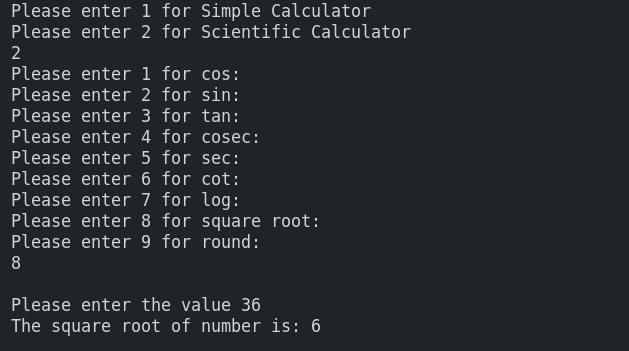Write a c++ program in which you are supposed to create two functions one for Simple Calculator and the other for Scientific Calculator and your program should perform tasks according to user choice either he/she wants to use Simple Calculator or Scientific Calculator and then it will perform certain operations as per need.c++ programming exercise with solution

Sample Input:

Please enter 1 for Simple Calculator

Please enter 2 for Scientific Calculator

2

8

Sample Output

The square root of number is: 6

#include <iostream>
#include <cmath>
using namespace std;

void SimpleCalculator()
{
int number1;
int number2;
char symbl;
cin>>number1;
cin>>number2;
cout<<"Please enter a symbol (+,-,/,*,%): ";
cin>>symbl;
switch (symbl)
{
case '+':
cout<<"The sum of two numbers is: "<<number1 + number2;
break;
case '-':
cout<<"The sum of two numbers is: "<<number1 - number2;
break;
case '*':
cout<<"The sum of two numbers is: "<<number1 * number2;
break;
case '/':
cout<<"The sum of two numbers is: "<<number1 / number2;
break;
case '%':
cout<<"The sum of two numbers is: "<<number1 % number2;
break;
default:
cout<<":<( Invalid.";
}
}

void ScientificCalculator()
{
int op;
float value;

cout<<"Please enter 1 for cos: \n";
cout<<"Please enter 2 for sin: \n";
cout<<"Please enter 3 for tan: \n";
cout<<"Please enter 4 for cosec: \n";
cout<<"Please enter 5 for sec: \n";
cout<<"Please enter 6 for cot: \n";
cout<<"Please enter 7 for log: \n";
cout<<"Please enter 8 for square root: \n";
cout<<"Please enter 9 for round: \n";

cin>>op;
cin>>value;
switch (op)
{
case 1:
cout<<"The value of cos: "<<cos(value);
break;
case 2:
cout<<"The value of sin: "<<sin(value);
break;
case 3:
cout<<"The value of tan: "<<tan(value);
break;
case 4:
cout<<"The value of cosec: "<<asin(value);
break;
case 5:
cout<<"The value of sec: "<<acos(value);
break;
case 6:
cout<<"The value of cot: "<<atan(value);
break;
case 7:
cout<<"The value of log: "<<log(value);
break;
case 8:
cout<<"The square root of number is: "<<sqrt(value);
break;
case 9:
cout<<"The rounded value is: "<<round(value);
break;
default:
cout<<":<( Invalid.";
}

}
int main()
{
int number;
cout<<"Please enter 1 for Simple Calculator\n";
cout<<"Please enter 2 for Scientific Calculator\n";
cin>>number;
if (number == 1)
{
SimpleCalculator();
}
else if (number == 2)
{
ScientificCalculator();
}
else
{
cout<<":<( Invalid.";
}
return 0;
}